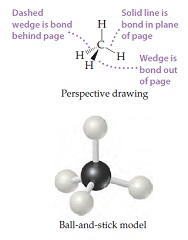# Problem: The methane molecule, CH4, has the geometry shown in following figure. Imagine a hypothetical process in which the methane molecule is "expanded," by simultaneously extending all four C–H bonds to infinity. We then have the process CH4(g) → C(g) + 4 H(g). Calculate the enthalpy change in process in which the methane molecule is expanded, by simultaneously extending all four C–H bonds to infinity.

###### FREE Expert Solution

We are asked to calculate the enthalpy change in process in which the methane molecule is expanded, by simultaneously extending all four C–H bonds to infinity.

Note that we need to multiply each ΔH˚ by the stoichiometric coefficient since ΔH˚ is in kJ/mol.

Balanced reaction : CH4(g) → C(g) + 4 H(g).

83% (369 ratings)###### Problem Details
The methane molecule, CH4, has the geometry shown in following figure. Imagine a hypothetical process in which the methane molecule is "expanded," by simultaneously extending all four C–H bonds to infinity. We then have the process CH4(g) → C(g) + 4 H(g). Calculate the enthalpy change in process in which the methane molecule is expanded, by simultaneously extending all four C–H bonds to infinity.Frequently Asked Questions

What scientific concept do you need to know in order to solve this problem?

Our tutors have indicated that to solve this problem you will need to apply the Enthalpy of Formation concept. You can view video lessons to learn Enthalpy of Formation. Or if you need more Enthalpy of Formation practice, you can also practice Enthalpy of Formation practice problems.